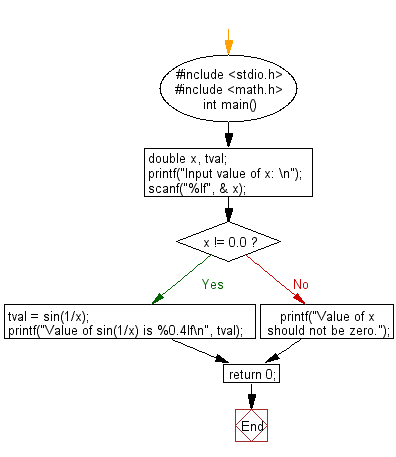﻿ C : Corresponding value of sin(1/x) using 4-decimal places

# C Exercises: Print out the corresponding value of sin(1/x) using 4-decimal places

## C Basic Declarations and Expressions: Exercise-61 with Solution

Write a C program that accepts a real number x and prints out the corresponding value of sin(1/x) using 4-decimal places.

Note: Use 4-decimal places.
Test data and expected output:
Input value of x:
Value of sin(1/x) is 0.8415

Sample Solution:

C Code:

``````#include <stdio.h>
#include <math.h>
int main() {
double x, tval;

// Prompt user for input
printf("Input value of x: \n");

scanf("%lf", &x);

if (x != 0.0) {
// Calculate sin(1/x) if x is not zero
tval = sin(1/x);
printf("Value of sin(1/x) is %0.4lf\n", tval);
} else {
// Display error message if x is zero
printf("Value of x should not be zero.");
}

return 0;
}
``````

Sample Output:

```Input value of x:
Value of sin(1/x) is 0.9995
```

Flowchart:C programming Code Editor:

What is the difficulty level of this exercise?

Test your Programming skills with w3resource's quiz.

﻿